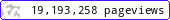•••••MadAsMaths.com :: Maths Booklets :: Elementary Topics

ELEMENTARY TOPICS

These booklets are suitable for

• able G.C.S.E students

• introductory work for A Level mathematics.

 basic_skills.pdfbasic_skills_student_version.pdfequations_basic_techniques.pdfpractical_arithmetic_problem_solving.pdfquadratics.pdf